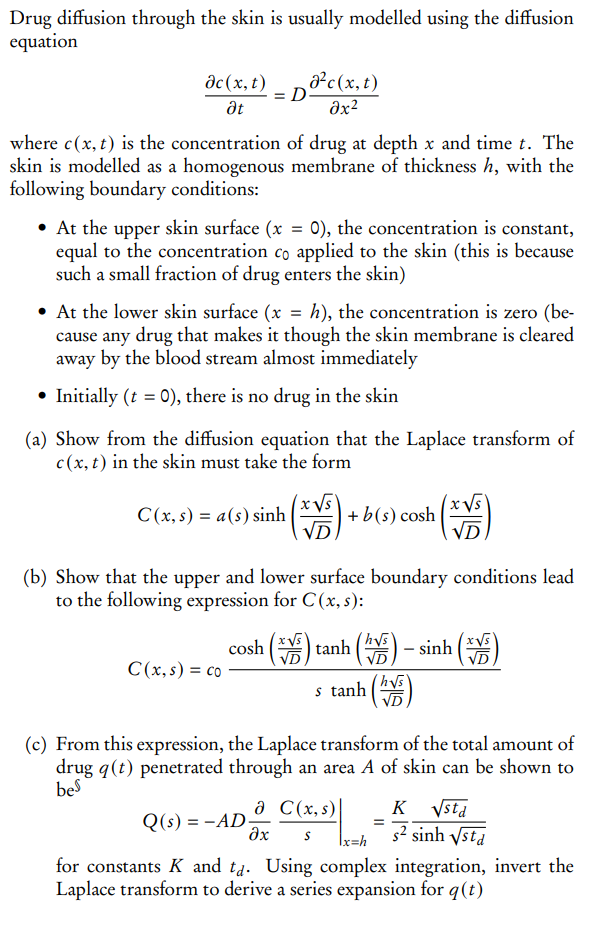# (Solved): Drug diffusion through the skin is usually modelled using the diffusion equation tc(x,t)=D ...Drug diffusion through the skin is usually modelled using the diffusion equation where is the concentration of drug at depth and time . The skin is modelled as a homogenous membrane of thickness , with the following boundary conditions: - At the upper skin surface , the concentration is constant, equal to the concentration applied to the skin (this is because such a small fraction of drug enters the skin) - At the lower skin surface , the concentration is zero (because any drug that makes it though the skin membrane is cleared away by the blood stream almost immediately - Initially , there is no drug in the skin (a) Show from the diffusion equation that the Laplace transform of in the skin must take the form (b) Show that the upper and lower surface boundary conditions lead to the following expression for : (c) From this expression, the Laplace transform of the total amount of drug penetrated through an area of skin can be shown to be for constants and . Using complex integration, invert the Laplace transform to derive a series expansion for

We have an Answer from Expert

(a) To find the Laplace transform of c(x,t), we will take the Laplace transform of both sides of the diffusion equation with respect to t, using the notation $\mathcal{L} \{f(t)\} = F(s)$ for the Laplace transform of f(t), to get:
$$\mathcal{L}\left\{\frac{\partial c(x,t)}{\partial t}\right\} = \mathcal{L}\left\{D \frac{\partial^2 c(x,t)}{\partial x^2}\right\}$$
$$s C(x,s) - c(x,0) = D \frac{\partial^2 C(x,s)}{\partial x^2}$$
Since we have the initial condition that there is no drug in the skin at t=0, we have $c(x,0)=0$, and thus: